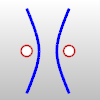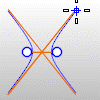# Hyperbola

The Hyperbola command draws a hyperbolic curve.

Steps

Follow the prompts for the selected option.

Command-line options

Default (Center)

The default option draws the hyperbola from the center, focus, and end.

Center steps

 1 Pick the center.
 2 Pick the focus.
 3 Pick the end. Your browser does not support the video tag.

FromCoefficient

The FromCoefficient option uses the A and B coefficients of the hyperbola equation to define the curve.

FromCoefficient steps

 1 Pick the center.
 2 Pick a direction.
 3 Pick the end.

FromCoefficient options

A/B

These are the A and B coefficients of the equation that defines the hyperbola. The A coefficient represents the distance from the center point to the vertex of the hyperbola. If C is the distance from the center point to the foci of the hyperbola, then B2 = C2 - A2. The B coefficient can be considered the slope of the asymptotes.

FromFoci

The FromFoci option draws the hyperbola starting from the focus points.

FromFoci steps

 1 Pick the first focus.
 2 Pick the second focus.
 3 Pick the end. Your browser does not support the video tag.

FromVertex

The FromVertex option draws the hyperbola starting from the vertices.

FromVertex steps

 1 Pick the vertex.
 2 Pick the focus.
 3 Pick the end. Your browser does not support the video tag.

Drawing and display options

MarkFoci=Yes/No

If Yes, a point object is placed at the focus locations.BothBranches=Yes/No

If Yes both branches of the hyperbola are drawn.ShowAsymptotes=Yes/No

If Yes, the asymptotes of the hyperbola are displayed as it is drawn.